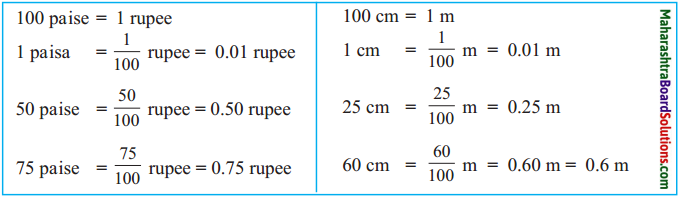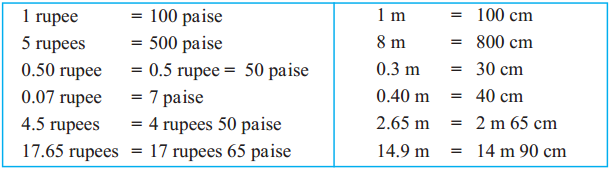Balbharti Maharashtra Board Class 5 Maths Solutions Chapter 9 Decimal Fractions Problem Set 38 Textbook Exercise Important Questions and Answers.

## Maharashtra State Board Class 5 Maths Solutions Chapter 9 Decimal Fractions Problem Set 38

Read the decimal fraction and write down the place value of each digit.

(1) 6.13
Six point one three.
Here 6 is in the units place. Hence, its place value is 6 x 1 = 6
1 is in tenths place. Hence, its place value is
$$1 \times \frac{1}{10}=0.1$$
3 is in the hundredths place. Its place value is 3 x $$\frac{1}{100}$$ = 0.03 100(2) 48.84
Fourty eight point eight four
Place value of 4 is 4 x 10 = 40 and of 8, it is 8 x 1 = 8
Place value of 8 is 8 x $$\frac{1}{10}=\frac{8}{10}$$ = 0.8 and
Place value of 4 is 4 x $$\frac{1}{100}=\frac{4}{100}$$ = 0.04

(3) 72.05
Seventy two point zero five
Place value of 7 is 7 x 10 = 70 and of 2 is, it is 2 x 1 = 2
Place value of 5 is 5 x $$\frac{1}{100}=\frac{5}{100}$$ = 0.05

(4) 3.4
Three point four.
Place value of 3 is 3 x 1 = 3
Place value of 4 is 4 x $$\frac{1}{10}$$ = 0.4(5) 0.59
Zero point five nine.
Place value of 5 is 5 x $$\frac{1}{10}=\frac{5}{10}$$ = 0.5 and
Place value of 9 is 9 x $$\frac{1}{100}=\frac{9}{100}$$ = 0.09

Use of decimal fractions

Sir : Now we will see how 24.50 equals 24 rupees and 50 paise. How many rupees is one paisa?
Sumit : 100 paise make one rupee, therefore, 1 paisa is one-hundredth of a rupee or 0.01 rupee.
Sir : And 50 paise are?
Sumit : 50 hundredths of a rupee, or 0.50 rupees, so 24.50 rupees is 24 rupees and 50 paise.
Sir : When a large unit of a certain quantity is divided into 10 or 100 parts to make smaller units, it is more convenient to write them in decimal form. As we just saw, 100 paise = 1 rupee. Similarly, 100 cm = 1 metre, so 75 cm = 0.75 m. 10 mm = 1 cm, so 1 mm = 0.1cm. 3 mm are 0.3 cm. 6.3 cm are 6 cm and 3 mm.

Now study the following table.(1) 12.34
Twelve point three four.
Place value of 1 is 1 x 10 = 10
Place value of 2 is 2 x 1 = 2
Place value of 3 is 3 x $$\frac{1}{10}$$ = 0.3
Place value of 4 is 4 x $$\frac{1}{100}$$ = 0.04(2) 369,58
Place value of 5, which is in the tenths place is 5 x $$\frac{1}{10}$$ = 0.5
Place value of 8, which is in the hundredths place is 8 x $$\frac{1}{100}$$ = 0.08
Place value of 5, which is in the tenths place is 5 x $$\frac{1}{10}$$ = 0.5